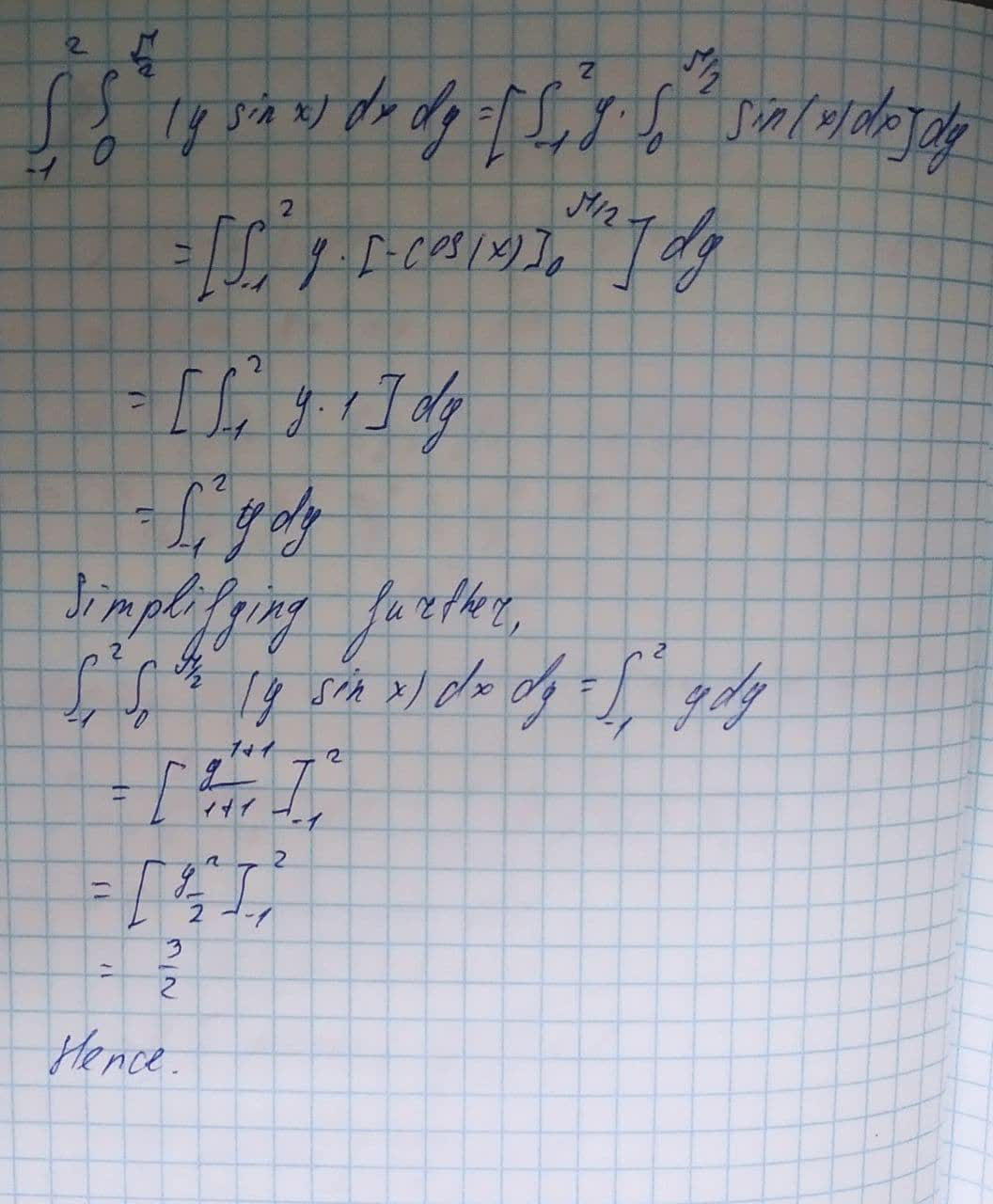Question# Evaluate the iterated integral \int_{-1}^{2}\int_{0}^{\frac{\pi}{2}}(y\sin x)dx dy

Applications of integrals
ANSWEREDEvaluate the iterated integral.
$$\displaystyle{\int_{{-{1}}}^{{{2}}}}{\int_{{{0}}}^{{{\frac{{\pi}}{{{2}}}}}}}{\left({y}{\sin{{x}}}\right)}{\left.{d}{x}\right.}{\left.{d}{y}\right.}$$